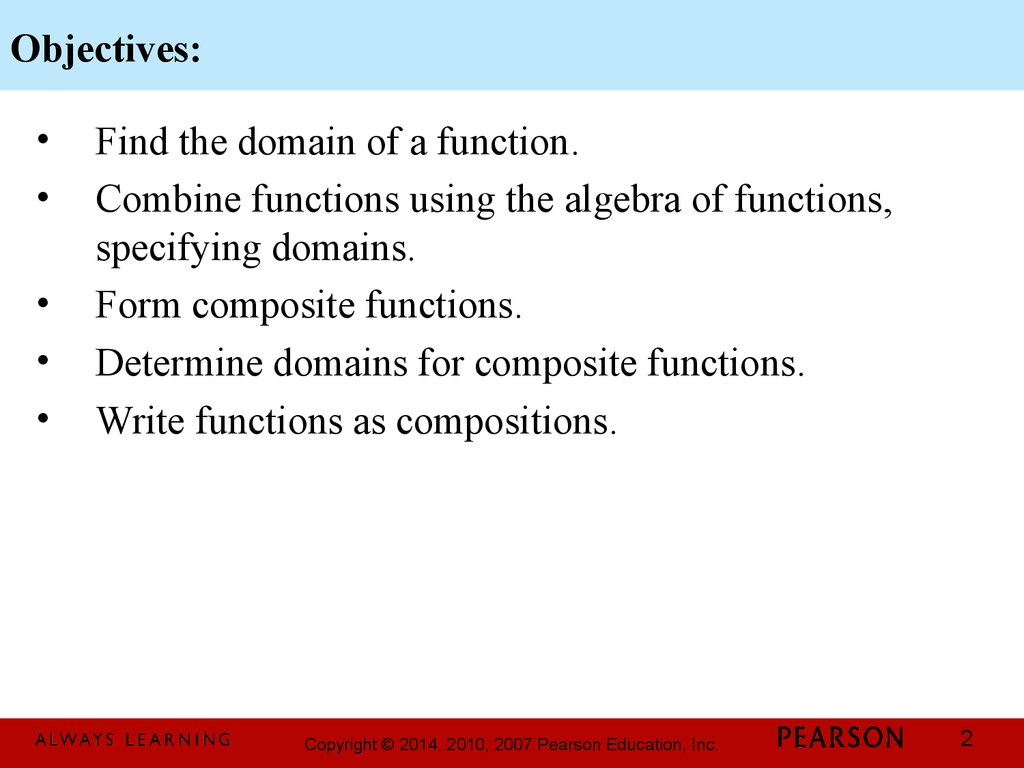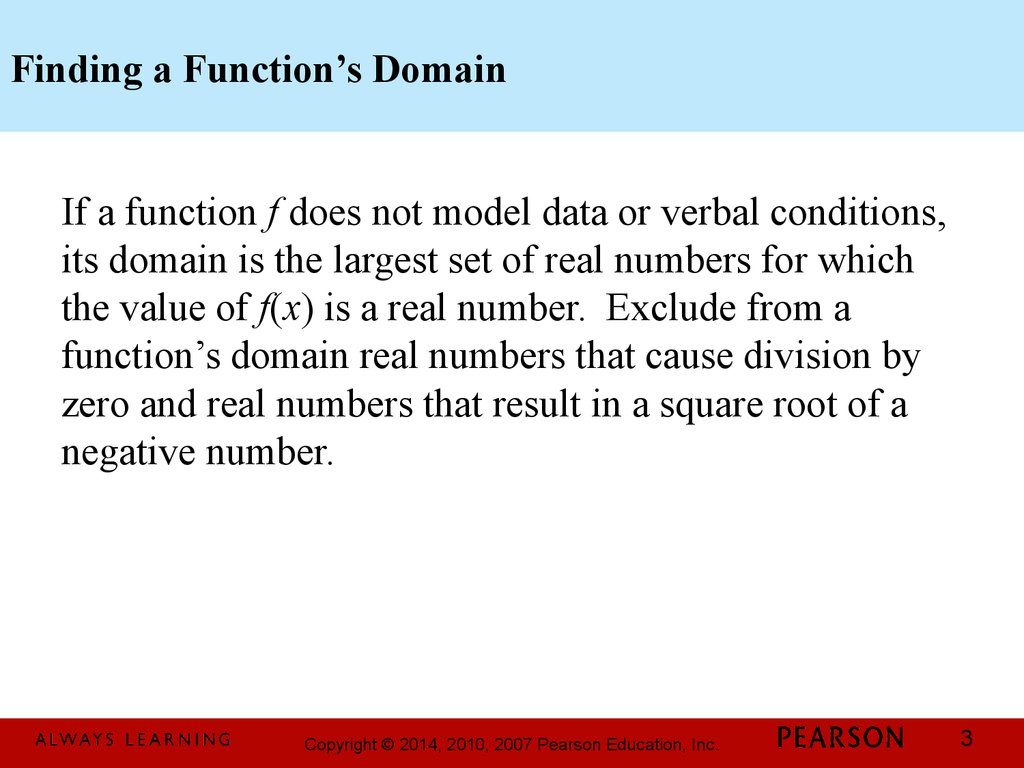# Functions and graphs. Chapter 2. Combinations of functions; composite functions

## 1.

Chapter 2
Functions and
Graphs
2.6 Combinations of
Functions; Composite
Functions
1

## 2. Objectives:

Find the domain of a function.
Combine functions using the algebra of functions,
specifying domains.
Form composite functions.
Determine domains for composite functions.
Write functions as compositions.
2

## 3. Finding a Function’s Domain

If a function f does not model data or verbal conditions,
its domain is the largest set of real numbers for which
the value of f(x) is a real number. Exclude from a
function’s domain real numbers that cause division by
zero and real numbers that result in a square root of a
negative number.
3

## 4. Example: Finding the Domain of a Function

Find the domain of the function
5x
g ( x) = 2
x - 49
Because division by 0 is undefined, we must exclude
from the domain the values of x that cause the
denominator to equal zero.
x 2 - 49 = 0
We exclude 7 and – 7 from
2
the domain of g.
x = 49
The domain of g is
x = ± 49
(-¥, -7) U (-7,7) U (7, ¥)
x = ±7
4

## 5. The Algebra of Functions: Sum, Difference, Product, and Quotient of Functions

Let f and g be two functions. The sum f + g, the
difference, f – g, the product fg, and the quotient f
are functions whose domains are the set of all realg
numbers common to the domains of f and g ( D f I Dg ),
defined as follows:
( f + g )( x) = f ( x) + g ( x)
1. Sum:
2. Difference: ( f - g )( x) = f ( x) - g ( x)
( fg )( x) = f ( x)gg ( x)
3. Product:
f ( x)
æfö
4. Quotient:
ç g ÷ ( x) = g ( x)
è ø
5

## 6. Example: Combining Functions

2
g
(
x
)
=
x
- 1. Find each of the
Let f ( x) = x - 5 and
following:
a. ( f + g )( x) = ( x - 5) + ( x 2 - 1) = x 2 + x - 6
b. The domain of ( f + g )( x)
The domain of f(x) has no restrictions.
The domain of g(x) has no restrictions.
The domain of ( f + g )( x) is (-¥, ¥)
6

## 7. The Composition of Functions

The composition of the function f with g is denoted f og
and is defined by the equation
( f og )( x) = f ( g ( x))
The domain of the composite function f og is the set
of all x such that
1. x is in the domain of g and
2. g(x) is in the domain of f.
7

## 8. Example: Forming Composite Functions

2
g
(
x
)
=
2
x
- x - 1, find f og
Given f ( x) = 5 x + 6 and
f og = f ( g ( x)) = f (2 x 2 - x - 1)
= 5(2 x 2 - x - 1) + 6
= 10 x 2 - 5 x - 5 + 6
= 10 x 2 - 5 x + 1
8

## 9. Excluding Values from the Domain of

( f og )( x) = f ( g ( x))
The following values must be excluded from the
input x:
If x is not in the domain of g, it must not be in the
domain of f og .
Any x for which g(x) is not in the domain of f must not
be in the domain of f og .
9

## 10. Example: Forming a Composite Function and Finding Its Domain

4
1
Given f ( x) =
and g ( x) =
x+2
x
Find ( f og )( x)
4

4 x
æ
( f og )( x) = f ( g ( x)) = f ç ÷ =
=
g
1
è xø 1 +2
+2 x
x
x
4
x
( f og )( x) = f ( g ( x)) =
1 + 2x
10

## 11. Example: Forming a Composite Function and Finding Its Domain

4
1
Given f ( x) =
and g ( x) =
x+2
x
Find the domain of ( f og )( x)
For g(x), x ¹ 0
4x
,
For ( f og )( x) =
1 + 2x
1
x¹2
1ö æ 1 ö
æ
The domain of ( f og )( x) is ç -¥, - ÷ U ç - ,0 ÷ U ( 0, ¥ )
2ø è 2 ø
è